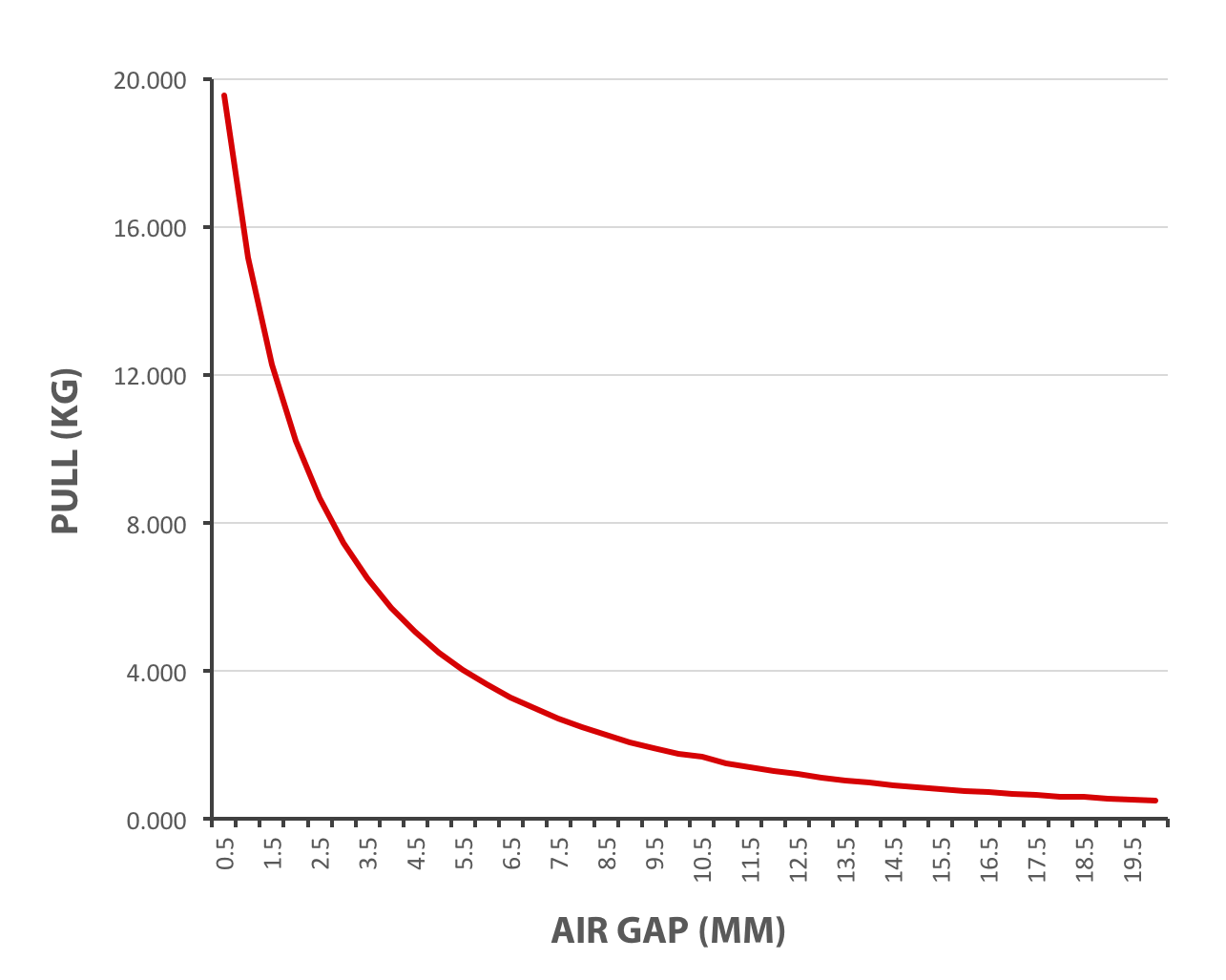# Explanations on Magnetic Pull Force & Calculating Equation | HVR MAGThere’s this question I saw on Internet: what is the scientific word for the pulling force of magnets? Therefore, I decided to write something about magnetic pull force, or magnet pull strength also called. This blog is an attempt to explain what magnetic pull force is, its synonyms and how to calculate it with equation.Before getting straight to the point, there’s another magnet related phrase that I’d like to point out – breakaway magnetic force. It refers to the force required to detach a magnet from a work load surface when the force is applied normal to the work load surface and through the center of force of the magnet.

Magnet pull strength is the highest possible holding power of a magnet, measured in kilograms. It is the force required to prise a magnet away from a flat steel surface when the magnet and metal have full and direct surface-to-surface contact. The grade of the metal, surface condition and angle of pull all have an impact on the pull strength.

Magnetic pull force equation/formula: F = m x a
F is the force required to move an object, m refers to the mass of the object, a stands for acceleration. So force = mass multiplied by acceleration.

Pull force testers are used to test the holding force of a magnet that is in contact with a flat steel plate. Pull forces are measured in pounds (or kilograms).

Influence Factor of Magnetic Pull Force

When we consider the ‘strength’ of a magnet, there are several measurements that all contribute to a magnet’s strength in different ways, which can often seem confusing. The size and shape of a magnet affects its magnetic field output and also its maximum possible pull force (holding force). Temperature, environmental conditions, the material being attracted (size, quality, shape, permeability, etc) all impact on the magnet performance, as does the distance between the magnet and the material being attracted.

It also depends on what is meant by strength; pulling force and magnetic field strength are often both summarised as strength.

MAXIMUM ENERGY PRODUCT
The maximum energy product of a magnet is measured in Mega Gauss Oersteds (MGOe).This is the primary indicator of a magnets ‘strength’. In general, the higher the maximum energy product value, the greater the magnetic field the magnet will generate in a particular application.
Maximum energy product, also referred to as BHmax is calculated by multiplying a magnet’s remanence(Br) and coercivity (Hc).

REMANENCE
Remanence, measured in Gauss, is described as the magnetism that is left in a magnet, after the removal of the external magnetic force applied to magnetise it. When a material has been magnetised, it has remanence, as the magnetism has at some point been induced by an external magnetic field.

COERCIVITY
The coercivity of a magnet is the energy required to reduce the magnetisation of a magnetised object to zero, which had previously been magnetised to the point of saturation. Essentially, it measures a magnetic material’s resistance to demagnetisation. The coercivity of magnetic material is measured in Oersteds(Oe).

PULL GAP CURVE
A pull-gap curve plots the pulling power of a magnet in direct contact with a thick and flat piece of steel and then through a steadily increasing range of air gaps. All magnets can be tested over a variety of air gaps using a pull-gap testing machine.Magnetic pull force, magnetic holding force, magnetic pull strength, magnetic breakaway force, these are all magnet related terms. As to magnets used for lifting, like lift magnets, lifting capacity is a standard measurement of their lifting force. HVR MAG designs and manufactures lifting magnets with a safety factor of 3:1, for various steel material handling applications.